﻿ “互联网+”公益模式对公益事业的影响研究——以杭州市为例

# “互联网+”公益模式对公益事业的影响研究——以杭州市为例The Influence of “Internet+” Public Welfare Mode on the Implementation of Public Welfare—A Case Study of Hangzhou

Abstract: This paper studied the influence of the “Internet+” public welfare model on the implementation of social public welfare in Hangzhou. With the data obtained from questionnaire survey, we first studied the positive and negative effects of “Internet+” mode on the implementation of social public welfare comparing to the traditional modes with the techniques of contingency table analysis and multi-value Logistic regression analysis; we then used confirmatory factor analysis and analytic hierarchy process to establish the “Internet+” quantitative evaluation model of public satisfaction, and some feasible suggestions were given in the last section of this paper.

1. 引言

2. 研究对象及研究方法

2.1. 研究对象

2.2. 研究方法

1) 文献资料法

2) 预调查

3)问卷调查法

3. 数据分析

3.1. 多值Logistic回归分析

3.1.1. 互联网+公益较传统公益的社会需求度

$\begin{array}{l}p\left(y=1\right)=\frac{{\text{e}}^{7.464+0.787{x}_{3}-0.926{x}_{4}-0.646{x}_{6}-0.849{x}_{9}}}{1+{\text{e}}^{7.464+0.787{x}_{3}-0.926{x}_{4}-0.646{x}_{6}-0.849{x}_{9}}+{\text{e}}^{6.764+0.723{x}_{3}-0.840{x}_{4}-0.734{x}_{6}-0.204{x}_{9}}+{\text{e}}^{-0.513-0.033{x}_{3}-0.632{x}_{4}+{x}_{6}+0.006{x}_{9}}}\\ p\left(y=2\right)=\frac{{\text{e}}^{6.764+0.723{x}_{3}-0.840{x}_{4}-0.734{x}_{6}-0.204{x}_{9}}}{1+{\text{e}}^{7.464+0.787{x}_{3}-0.926{x}_{4}-0.646{x}_{6}-0.849{x}_{9}}+{\text{e}}^{6.764+0.723{x}_{3}-0.840{x}_{4}-0.734{x}_{6}-0.204{x}_{9}}+{\text{e}}^{-0.513-0.033{x}_{3}-0.632{x}_{4}+{x}_{6}+0.006{x}_{9}}}\\ p\left(y=3\right)=\frac{{\text{e}}^{-0.513-0.033{x}_{3}-0.632{x}_{4}+{x}_{6}+0.006{x}_{9}}}{1+{\text{e}}^{7.464+0.787{x}_{3}-0.926{x}_{4}-0.646{x}_{6}-0.849{x}_{9}}+{\text{e}}^{6.764+0.723{x}_{3}-0.840{x}_{4}-0.734{x}_{6}-0.204{x}_{9}}+{\text{e}}^{-0.513-0.033{x}_{3}-0.632{x}_{4}+{x}_{6}+0.006{x}_{9}}}\\ p\left(y=4\right)=\frac{1}{1+{\text{e}}^{7.464+0.787{x}_{3}-0.926{x}_{4}-0.646{x}_{6}-0.849{x}_{9}}+{\text{e}}^{6.764+0.723{x}_{3}-0.840{x}_{4}-0.734{x}_{6}-0.204{x}_{9}}+{\text{e}}^{-0.513-0.033{x}_{3}-0.632{x}_{4}+{x}_{6}+0.006{x}_{9}}}\end{array}$

3.1.2. 互联网+公益对社会公益事业的影响

1) 积极影响：

$\begin{array}{l}p\left(y=1\right)=\frac{{\text{e}}^{0.963+0.059{x}_{2}+0.451{x}_{3}+0.123{x}_{6}}}{1+{\text{e}}^{0.963+0.059{x}_{2}+0.451{x}_{3}+0.123{x}_{6}}+{\text{e}}^{2.475-0.328{x}_{2}-0.013{x}_{3}+0.291{x}_{6}}+{\text{e}}^{1.526-0.018{x}_{2}+0.168{x}_{3}-0.602{x}_{6}}}\\ p\left(y=2\right)=\frac{{\text{e}}^{2.475-0.328{x}_{2}-0.013{x}_{3}+0.291{x}_{6}}}{1+{\text{e}}^{0.963+0.059{x}_{2}+0.451{x}_{3}+0.123{x}_{6}}+{\text{e}}^{2.475-0.328{x}_{2}-0.013{x}_{3}+0.291{x}_{6}}+{\text{e}}^{1.526-0.018{x}_{2}+0.168{x}_{3}-0.602{x}_{6}}}\\ p\left(y=3\right)=\frac{{\text{e}}^{1.526-0.018{x}_{2}+0.168{x}_{3}-0.602{x}_{6}}}{1+{\text{e}}^{0.963+0.059{x}_{2}+0.451{x}_{3}+0.123{x}_{6}}+{\text{e}}^{2.475-0.328{x}_{2}-0.013{x}_{3}+0.291{x}_{6}}+{\text{e}}^{1.526-0.018{x}_{2}+0.168{x}_{3}-0.602{x}_{6}}}\\ p\left(y=4\right)=\frac{1}{1+{\text{e}}^{0.963+0.059{x}_{2}+0.451{x}_{3}+0.123{x}_{6}}+{\text{e}}^{2.475-0.328{x}_{2}-0.013{x}_{3}+0.291{x}_{6}}+{\text{e}}^{1.526-0.018{x}_{2}+0.168{x}_{3}-0.602{x}_{6}}}\end{array}$

2) 消极影响：

$\begin{array}{l}p\left(y=1\right)=\frac{{\text{e}}^{10.623-2.585{x}_{2}-0.571{x}_{3}+1.538{x}_{6}}}{1+{\text{e}}^{10.623-2.585{x}_{2}-0.571{x}_{3}+1.538{x}_{6}}+{\text{e}}^{11.538-2.221{x}_{2}-0.757{x}_{3}+0.975{x}_{6}}+{\text{e}}^{11.814-2.499{x}_{2}-0.964{x}_{3}+1.006{x}_{6}}}\\ p\left(y=2\right)=\frac{{\text{e}}^{11.538-2.221{x}_{2}-0.757{x}_{3}+0.975{x}_{6}}}{1+{\text{e}}^{10.623-2.585{x}_{2}-0.571{x}_{3}+1.538{x}_{6}}+{\text{e}}^{11.538-2.221{x}_{2}-0.757{x}_{3}+0.975{x}_{6}}+{\text{e}}^{11.814-2.499{x}_{2}-0.964{x}_{3}+1.006{x}_{6}}}\\ p\left(y=3\right)=\frac{{\text{e}}^{11.814-2.499{x}_{2}-0.964{x}_{3}+1.006{x}_{6}}}{1+{\text{e}}^{10.623-2.585{x}_{2}-0.571{x}_{3}+1.538{x}_{6}}+{\text{e}}^{11.538-2.221{x}_{2}-0.757{x}_{3}+0.975{x}_{6}}+{\text{e}}^{11.814-2.499{x}_{2}-0.964{x}_{3}+1.006{x}_{6}}}\\ p\left(y=4\right)=\frac{1}{1+{\text{e}}^{10.623-2.585{x}_{2}-0.571{x}_{3}+1.538{x}_{6}}+{\text{e}}^{11.538-2.221{x}_{2}-0.757{x}_{3}+0.975{x}_{6}}+{\text{e}}^{11.814-2.499{x}_{2}-0.964{x}_{3}+1.006{x}_{6}}}\end{array}$

3.2. 基于验证性因子分析和层次分析构建满意度评价指标

3.2.1. 数据检验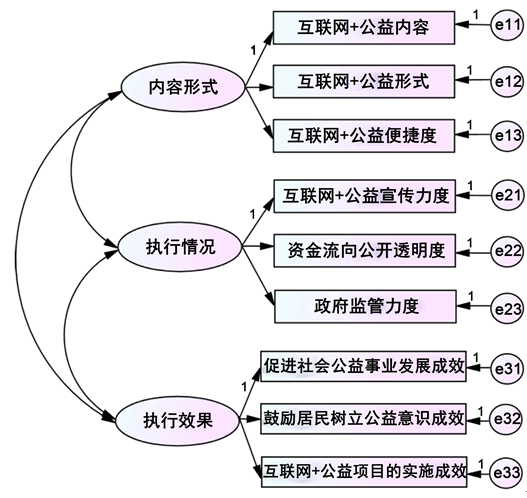Figure 1. AMOS verification factor analysis model schematic diagramTable 1. List of model fitting parameters

3.2.2. 基于层次分析法确定各级指标权重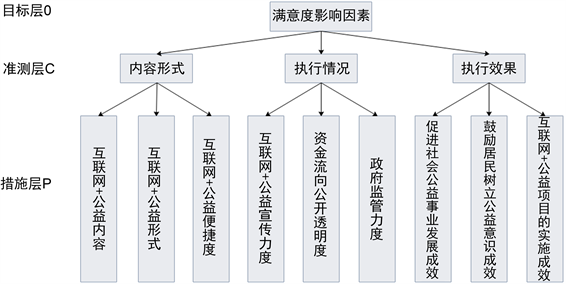Figure 2. Tree diagram of satisfaction evaluation system

$A=\left(\begin{array}{ccc}1& 3& 2\\ 1/3& 1& 1/2\\ 1/2& 2& 1\end{array}\right)$

$\lambda =3.0092,\omega ={\left({\omega }_{1},{\omega }_{2},{\omega }_{3}\right)}^{\text{T}}={\left(0.8468,0.2565,0.4660\right)}^{\text{T}}$

$CI=0.0046,CR=0.0088<0.1$ .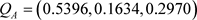.,,.

Table 2. The result table is the judgment matrix of measure layer to criterion layerTable 3. Model fitting parameter list Hangzhou residents “Internet+ public welfare” model satisfaction evaluation system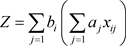3.2.3. 不同影响因素对满意度的作用情况分析

1) 不同年龄段居民对“互联网+公益”模式满意度的差异性分析Table 4. Score table of Hangzhou residents of different age groups on the second-level index of satisfaction with “Internet+ public welfare” mode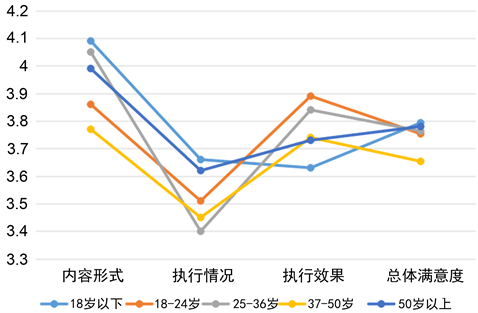Figure 3. The score chart of Hangzhou residents' satisfaction towards “Internet+ public welfare” mode in different age groups

2) 不同文化程度居民对“互联网+公益”模式满意度的差异性分析Table 5. Score table of Hangzhou residents with different educational levels on the satisfaction level of “Internet+ public welfare” mode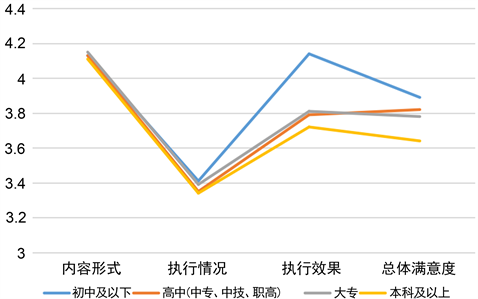Figure 4. Score chart of Hangzhou residents’ satisfaction towards “Internet+ public welfare” mode with different educational levels

4. 研究结果及启示

1) 加强政府监管

2) 加强信息透明化与完善化

3) 增强居民持续关注公益项目的意识

4) 加大宣传力度

NOTES

*通讯作者。

 王荣. 如何确定样本量[J]. 北京统计, 2002(7): 43-44.

 早教700. “互联网 + 民政”: 引领新时代智慧民政发展应用研究[[Z/OL].
https://www.zj700.com/jingjifalv/2019/0929/845579.html, 2019-09-29.

 徐静远. 抽样调查中样本量的确定[J]. 统计与咨询, 2009(4): 43.

 谷恒明, 胡良平. 经典统计的回归模型概述[J]. 四川精神卫生, 2017, 30(6): 489-493.

 Sharkey, C.M., Perez, M.N., Bakula, D.M., Grant, D.M. and Mullins, L.L. (2019) Exploratory Factor Analysis of the Mishel Uncertainty in Illness Scale Among Adolescents and Young Adults with Chronic Medical Conditions. Journal of Pediatric Health Care: Official Publication of National Association of Pediatric Nurse Associates & Practitioners, 33, 186-194.
https://doi.org/10.1016/j.pedhc.2018.08.002

 Hussein, R., Hajar, M.S., Cheng, K.Y., Kueh, Y.C., Muhamad, A.S. and Kuan, G. (2019) Confirmatory Factor Analysis of the Malay-Language Transtheoretical Model of Physical Activity among Malaysian Primary School Children. The Malaysian Journal of Medical Sciences: MJMS, 26, 99-113.
https://doi.org/10.21315/mjms2019.26.2.11

Top